• +91 9971497814
• info@interviewmaterial.com

### Related Subjects

Question 1 :

Solving the following quadratic equations by factorization method:

(i) x2 + 10ix – 21 = 0

(ii) x2 + (1 – 2i)x – 2i = 0

(iii) x2 – (2√3 + 3i) x + 6√3i = 0

(iv) 6x2 – 17ix – 12 = 0

(i) x2 +10ix – 21 = 0

Given: x2 +10ix – 21 = 0

x2 +10ix – 21 × 1 = 0

We know, i2 =–1  1 = –i2

By substituting 1 = –i2 inthe above equation, we get

x2 +10ix – 21(–i2) = 0

x2 +10ix + 21i2 = 0

x2 +3ix + 7ix + 21i2 = 0

x(x + 3i) + 7i(x + 3i)= 0

(x + 3i) (x + 7i) = 0

x + 3i = 0 or x + 7i =0

x = –3i or –7i

The roots of thegiven equation are –3i, –7i

(ii) x2 +(1 – 2i)x – 2i = 0

Given: x2 +(1 – 2i)x – 2i = 0

x2 + x– 2ix – 2i = 0

x(x + 1) – 2i(x + 1) =0

(x + 1) (x – 2i) = 0

x + 1 = 0 or x – 2i =0

x = –1 or 2i

The roots of thegiven equation are –1, 2i

(iii) x2 –(2√3 + 3i) x + 6√3i = 0

Given: x2 –(2√3 + 3i) x + 6√3i = 0

x2 –(2√3x + 3ix) + 6√3i = 0

x2 –2√3x – 3ix + 6√3i = 0

x(x – 2√3) – 3i(x –2√3) = 0

(x – 2√3) (x – 3i) = 0

(x – 2√3) = 0 or (x –3i) = 0

x = 2√3 or x = 3i

The roots of thegiven equation are 2√3, 3i

(iv) 6x2 –17ix – 12 = 0

Given: 6x2 –17ix – 12 = 0

6x2 –17ix – 12 × 1 = 0

We know, i2 =–1  1 = –i2

By substituting 1 = –i2 inthe above equation, we get

6x2 –17ix – 12(–i2) = 0

6x2 –17ix + 12i2 = 0

6x2 –9ix – 8ix + 12i2 = 0

3x(2x – 3i) – 4i(2x –3i) = 0

(2x – 3i) (3x – 4i) =0

2x – 3i = 0 or 3x – 4i= 0

2x = 3i or 3x = 4i

x = 3i/2 or x = 4i/3

The roots of thegiven equation are 3i/2, 4i/3

Question 2 :

(i) x2 – (3√2 + 2i) x + 6√2i = 0

(ii) x2 – (5 – i) x + (18 + i) = 0

(iii) (2 + i)x2 – (5- i)x + 2 (1 – i) = 0

(iv) x2 – (2 + i)x – (1 – 7i) = 0

(v) ix2 – 4x – 4i = 0

(vi) x2 + 4ix – 4 = 0

(vii) 2x2 + √15ix – i = 0

(viii) x2 – x + (1 + i) = 0

(ix) ix2 – x + 12i = 0

(x) x2 – (3√2 – 2i)x – √2i = 0

(xi) x2 – (√2 + i)x + √2i = 0

(xii) 2x2 – (3 + 7i)x + (9i – 3) = 0

(i) x2 –(3√2 + 2i) x + 6√2i = 0

Given: x2 –(3√2 + 2i) x + 6√2i = 0

x2 –(3√2x + 2ix) + 6√2i = 0

x2 –3√2x – 2ix + 6√2i = 0

x(x – 3√2) – 2i(x –3√2) = 0

(x – 3√2) (x – 2i) = 0

(x – 3√2) = 0 or (x –2i) = 0

x = 3√2 or x = 2i

The roots of thegiven equation are 3√2, 2i

(ii) x2 –(5 – i) x + (18 + i) = 0

Given: x2 –(5 – i) x + (18 + i) = 0

We shall applydiscriminant rule,

Where, x = (-b ±√(b2 – 4ac))/2a

Here, a = 1, b =-(5-i), c = (18+i)

So,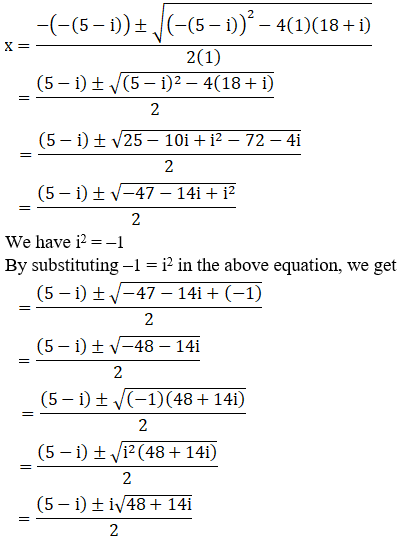We can write 48 + 14i= 49 – 1 + 14i

So,

48 + 14i = 49 + i2 +14i [ i2 =–1]

= 72 +i2 + 2(7)(i)

= (7 + i)2 [Since, (a+ b)2 = a2 + b2 + 2ab]

By using the result 48+ 14i = (7 + i) 2, we get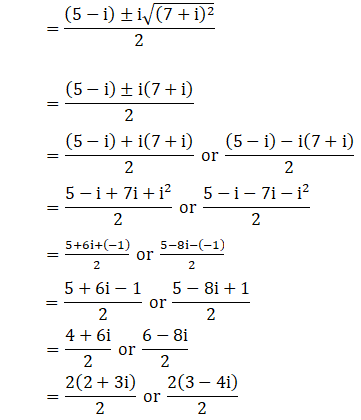x = 2 + 3i or 3 – 4i

The roots of thegiven equation are 3 – 4i, 2 + 3i

(iii) (2 + i)x2 –(5- i)x + 2 (1 – i) = 0

Given: (2 + i)x2 –(5- i)x + 2 (1 – i) = 0

We shall applydiscriminant rule,

Where, x = (-b ±√(b2 – 4ac))/2a

Here, a = (2+i), b =-(5-i), c = 2(1-i)

So,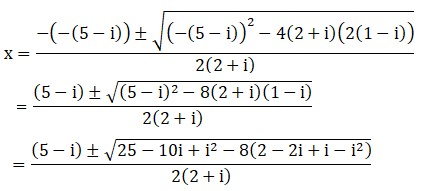We have i2 =–1

By substituting –1 = i2 inthe above equation, we get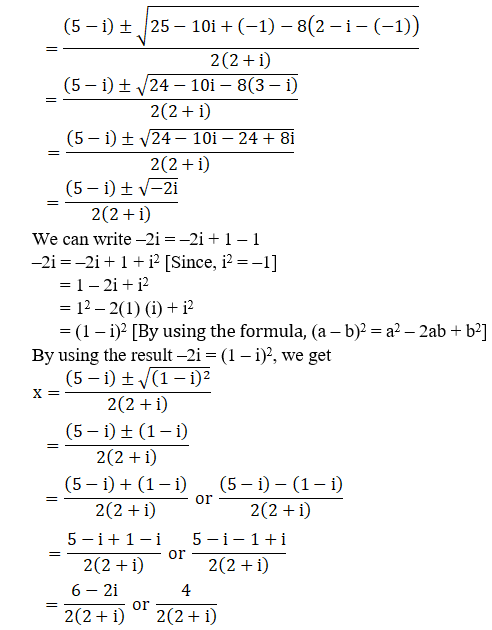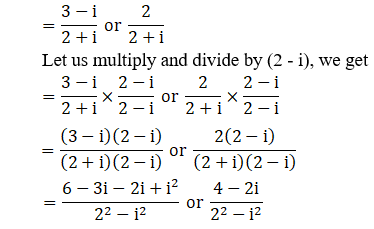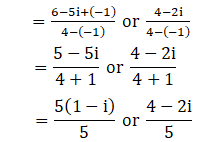x = (1 – i) or 4/5 –2i/5

The roots of thegiven equation are (1 – i), 4/5 – 2i/5

(iv) x2 –(2 + i)x – (1 – 7i) = 0

Given: x2 –(2 + i)x – (1 – 7i) = 0

We shall applydiscriminant rule,

Where, x = (-b ±√(b2 – 4ac))/2a

Here, a = 1, b =-(2+i), c = -(1-7i)

So,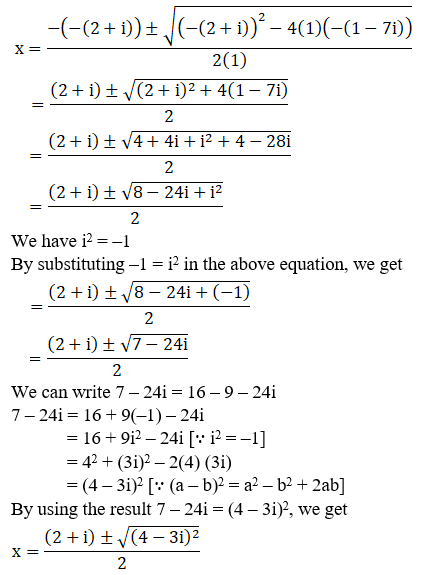We can write 7 – 24i =16 – 9 – 24i

7 – 24i = 16 + 9(–1) –24i

= 16 + 9i2 –24i [ i2 =–1]

= 42 +(3i)2 – 2(4) (3i)

= (4 – 3i)2 [ (a – b)2 = a2 –b2 + 2ab]

By using the result 7– 24i = (4 – 3i)2, we get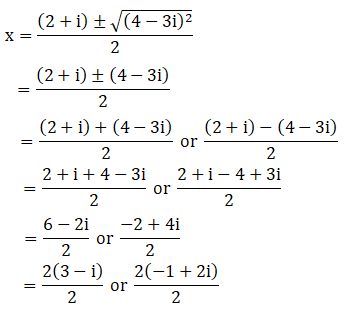x = 3 – i or -1 + 2i

The roots of thegiven equation are (-1 + 2i), (3 – i)

(v) ix2 –4x – 4i = 0

Given: ix2 –4x – 4i = 0

ix2 +4x(–1) – 4i = 0 [We know, i2 = –1]

So by substituting –1= i2 in the above equation, we get

ix2 +4xi2 – 4i = 0

i(x2 +4ix – 4) = 0

x2 +4ix – 4 = 0

x2 +4ix + 4(–1) = 0

x2 +4ix + 4i2 = 0 [Since, i2 = –1]

x2 +2ix + 2ix + 4i2 = 0

x(x + 2i) + 2i(x + 2i)= 0

(x + 2i) (x + 2i) = 0

(x + 2i)2 =0

x + 2i = 0

x = –2i, -2i

The roots of thegiven equation are –2i, –2i

(vi) x2 +4ix – 4 = 0

Given: x2 +4ix – 4 = 0

x2 +4ix + 4(–1) = 0 [We know, i2 = –1]

So by substituting –1= i2 in the above equation, we get

x2 +4ix + 4i2 = 0

x2 +2ix + 2ix + 4i2 = 0

x(x + 2i) + 2i(x + 2i)= 0

(x + 2i) (x + 2i) = 0

(x + 2i)2 =0

x + 2i = 0

x = –2i, -2i

The roots of thegiven equation are –2i, –2i

(vii) 2x2 +√15ix – i = 0

Given: 2x2 +√15ix – i = 0

We shall applydiscriminant rule,

Where, x = (-b ±√(b2 – 4ac))/2a

Here, a = 2, b = √15i,c = -i

So,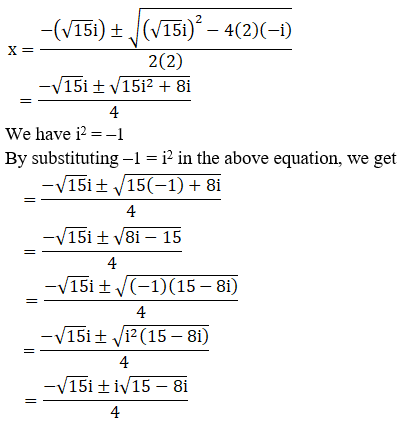We can write 15 – 8i =16 – 1 – 8i

15 – 8i = 16 + (–1) –8i

= 16 + i2 –8i [ i2 =–1]

= 42 +(i)2 – 2(4)(i)

= (4 – i)2 [Since, (a– b)2 = a2 – b2 + 2ab]

By using the result 15– 8i = (4 – i)2, we get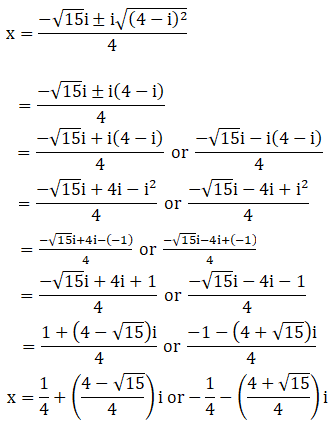The roots of thegiven equation are [1+ (4 – √15)i/4] , [-1 -(4 + √15)i/4]

(viii) x2 –x + (1 + i) = 0

Given: x2 –x + (1 + i) = 0

We shall applydiscriminant rule,

Where, x = (-b ±√(b2 – 4ac))/2a

Here, a = 1, b = -1, c= (1+i)

So,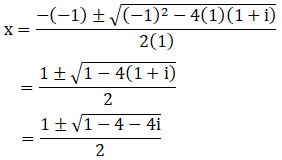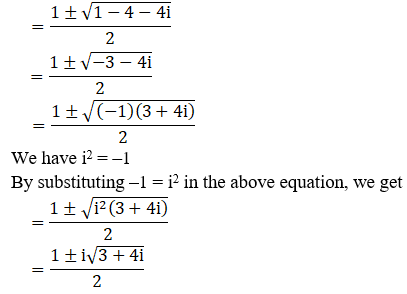We can write 3 + 4i =4 – 1 + 4i

3 + 4i = 4 + i2 +4i [ i2 =–1]

= 22 +i2 + 2(2) (i)

= (2 + i)2 [Since, (a+ b)2 = a2 + b2 + 2ab]

By using the result 3+ 4i = (2 + i)2, we get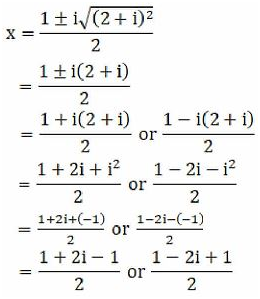x = 2i/2 or (2 – 2i)/2

x = i or 2(1-i)/2

x = i or (1 – i)

The roots of thegiven equation are (1-i), i

(ix) ix2 –x + 12i = 0

Given: ix2 –x + 12i = 0

ix2 +x(–1) + 12i = 0 [We know, i2 = –1]

so by substituting –1= i2 in the above equation, we get

ix2 +xi2 + 12i = 0

i(x2 +ix + 12) = 0

x2 +ix + 12 = 0

x2 +ix – 12(–1) = 0

x2 +ix – 12i2 = 0 [Since, i2 = –1]

x2 –3ix + 4ix – 12i2 = 0

x(x – 3i) + 4i(x – 3i)= 0

(x – 3i) (x + 4i) = 0

x – 3i = 0 or x + 4i =0

x = 3i or –4i

The roots of thegiven equation are -4i, 3i

(x) x2 –(3√2 – 2i)x – √2i = 0

Given: x2 –(3√2 – 2i)x – √2i = 0

We shall applydiscriminant rule,

Where, x = (-b ±√(b2 – 4ac))/2a

Here, a = 1, b = -(3√2 – 2i), c = –√2i

So,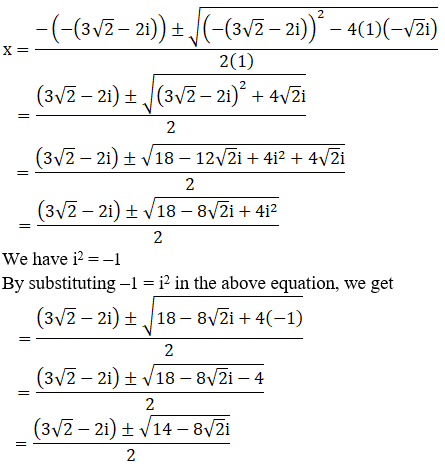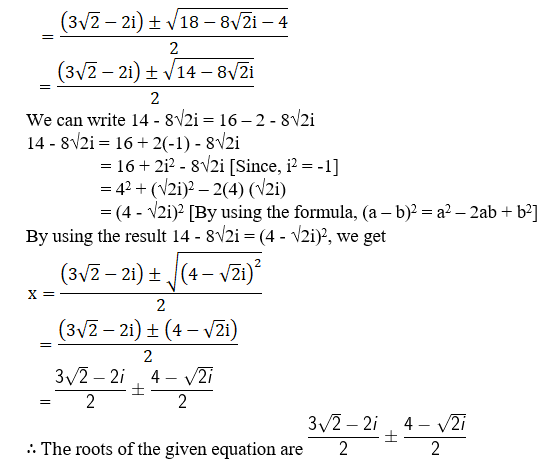(xi) x2 –(√2 + i)x + √2i = 0

Given: x2 –(√2 + i)x + √2i = 0

x2 – (√2x + ix) + √2i = 0

x2 – √2x – ix + √2i = 0

x(x – √2) – i(x – √2) = 0

(x – √2) (x – i) = 0

(x – √2) = 0 or (x – i) = 0

x = √2 or x = i

The roots of thegiven equation are i, √2

(xii) 2x2 –(3 + 7i)x + (9i – 3) = 0

Given: 2x2 –(3 + 7i)x + (9i – 3) = 0

We shall applydiscriminant rule,

Where, x = (-b ±√(b2 – 4ac))/2a

Here, a = 2, b =-(3 + 7i), c = (9i – 3)

So,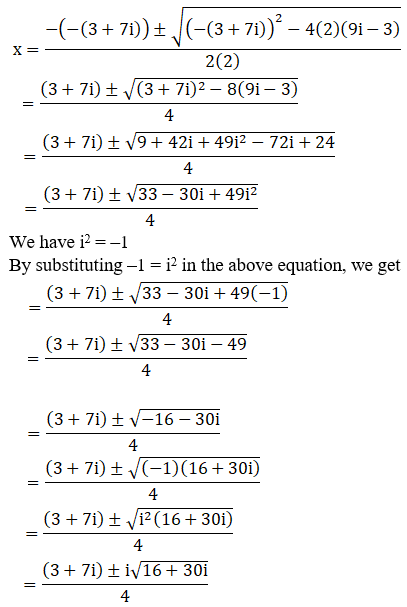We can write 16 + 30i= 25 – 9 + 30i

16 + 30i = 25 + 9(–1)+ 30i

= 25 + 9i2 +30i [ i2 =–1]

= 52 +(3i)2 + 2(5)(3i)

= (5 + 3i)2 [ (a + b)2 = a2 +b2 + 2ab]

By using the result 16+ 30i = (5 + 3i)2, we get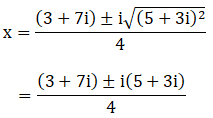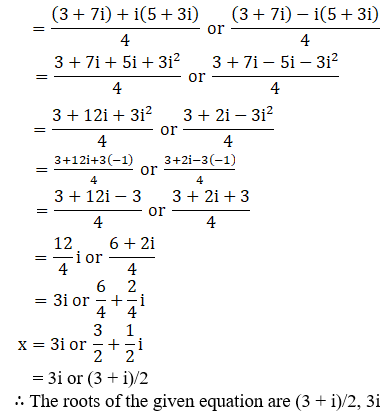Todays Deals### RD Chapter 14- Quadratic Equations Ex-14.2 Contributorskrishan

Name:
Email:

# Latest News# 9000 interview questions in different categories